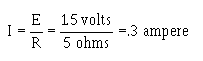voltage, and resistance. This relationship is called Ohm's law and is stated as follows: The current in a circuit is DIRECTLY proportional to the applied voltage and INVERSELY proportional to the circuit resistance. Ohm's law may be expressed as an equation:"> Ohm's lawCustom SearchThe basic electric circuitMatter, Energy, and ElectricityApplication of ohm's lawOHM'S LAW

In the early part of the 19th century, George Simon Ohm proved by experiment that a precise relationship exists between current, voltage, and resistance. This relationship is called Ohm's law and is stated as follows:

The current in a circuit is DIRECTLY proportional to the applied voltage and INVERSELY proportional to the circuit resistance. Ohm's law may be expressed as an equation:As stated in Ohm's law, current is inversely proportional to resistance. This means, as the resistance in a circuit increases, the current decreases proportionately.

In the equationif any two quantities are known, the third one can be determined. Refer to figure 3-2(B), the schematic of the flashlight. If the battery (BAT) supplies a voltage of 1.5 volts and the lamp (DS1) has a resistance of 5 ohms, then the current in the circuit can be determined. Using this equation and substituting values:If the flashlight were a two-cell flashlight, we would have twice the voltage, or 3.0 volts, applied to the circuit. Using this voltage in the equation:You can see that the current has doubled as the voltage has doubled.
This demonstrates that the current is directly proportional to the applied voltage.

If the value of resistance of the lamp is doubled, the equation will be:The current has been reduced to one half of the value of the previous equation, or .3 ampere. This demonstrates that the current is inversely proportional to the resistance. Doubling the value of the resistance of the load reduces circuit current value to one half of its former value.Integrated Publishing, Inc. - A (SDVOSB) Service Disabled Veteran Owned Small Business